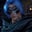Related Tags

python

# How to square a number in PythonGutha Vamsi Krishna

### Overview

We'll learn how to square a number in Python. We can achieve this using a multiplication operator, an inbuilt function, or using an exponent operator.

### Using the multiplication operator

We can get the square of a number by multiplying the number by itself.

### Code

#given number
num = 10
#square of given number
print(num*num)
Square of a number using multiplication operator

### Explanation

• Line 2: We declare and initialize the number.
• Line 4: We get the square of the given number and display it.

### Using the math.pow() method

We can get the square of a number using the pow() method provided by the math module. We'll pass the number to the first parameter and 2 to the second parameter to get the square of the number.

### Code

import math
#given number
num = 10
#get square of a number
print(math.pow(10,2))
Square of a number using math.pow() method

### Explanation

• Line 1: We import the module math, which provides the pow() method.
• Line 3: We declare the number and initialize it.
• Line 5: We use the pow() method to get the square of the given number, num, by passing the number num as the first parameter and 2 as the second parameter, and then we'll print the returned result.

### Using the exponent operator

We can get the square of a number in python using the exponent operator(**) if we provide the exponent value as 2.

### Code

#given number
num = 10
#square of given number using exponent operator
print(num**2)
Square of a number using exponent operator

### Explanation

• Line 2: We declare and initialize the number.
• Line 4: We get the square of the given number using the exponent operator where the exponent value is 2 and display it.

RELATED TAGS

python

CONTRIBUTORGutha Vamsi Krishna
RELATED COURSES

View all Courses

Keep Exploring

Learn in-demand tech skills in half the time